Select Page

# Seminar in spectral theory and related topics

These days seminar sessions are zoom meetings each Tuesday. To participate in the seminar, join zoom channel 812-916-426. If you do not know the password, please write an email to Roman Bessonov. To subscribe for seminar anonucements, please visit this page. Some records of talks given on the seminar are  avaliable here.

### I. Vergara, EIMI

Positive definite radial kernels on trees and products

A classical result in harmonic analysis on trees characterises positive definite radial kernels on a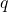-homogeneous tree (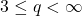) as integrals on the interval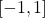of a certain family of polynomials related to the Laplace operator on the tree. This gives a one-to-one correspondence between such kernels and finite Borel measures on. I will present an extension of this result to finite products of homogeneous trees. The main tools used in the proof come from the theory of Schur multipliers and the Hamburger moment problem.

### P. Exner, Doppler Institute for Mathematical Physics and Applied Mathematics

Spectral properties of spiral quantum waveguides

We discuss properties of a particle confined to a spiral-shaped region with Dirichlet boundary. As a case study we analyze in detail the Archimedean spiral for which the spectrum above the continuum threshold is absolutely continuous away from the thresholds. The subtle difference between the radial and perpendicular width implies, however, that in contrast to ‘less curved’ waveguides, the discrete spectrum is empty in this case. We also discuss modifications such a multi-arm Archimedean spirals and spiral waveguides with a central cavity; in the latter case bound state already exist if the cavity exceeds a critical size. For more general spiral regions the spectral nature depends on whether they are ‘expanding’ or ‘shrinking’. The most interesting situation occurs in the asymptotically Archimedean case where the existence of bound states depends on the direction from which the asymptotics is reached.

### D. Borisov, Bashkir State Pedagogical University and Institute o Mathematics UFRC RAS

Accumulation of resonances and eigenvalues for operators with distant perturbations

We consider a model one-dimensional problem with distant perturbations, for which we study a phenomenon of emerging of infinitely many eigenvalues and resonances near the bottom of the essential spectrum. We show that they accumulate to a certain segment of the essential spectrum. Then we discuss possible generalization of this result to multi-dimensional models and various situations of resonances and eigenvalues distributions.

### K. Pankrashkin, Carl von Ossietzky University of Oldenburg

Some convergence results for Dirac operators with large parameters

We consider Euclidean Dirac operators with piecewise constant mass potentials and investigate their spectra in several asymptotic regimes in which the mass becomes large in some regions. If the mass jumps along a smooth interface, then it appears that the (low-lying) discrete spectrum of such an operator converges to the (low-lying) discrete spectrum of an effective operator acting either on or in the interior of the interface. The effective operators admit a simple geometric interpretation in terms of the spin geometry, and the results can be extended to a class of spin manifolds as well. Most questions remain open if the jump interface is non-smooth. Based on joint works with Brice Flamencourt, Markus Holzmann, Andrei Moroianu, and Thomas Ourmieres-Bonafos.

TBA

TBA

## A new complex frequency spectrum for the analysis of transmission efficiency in waveguide-like geometries

We consider a waveguide, with one inlet and one outlet, and some arbitrary perturbation in between. In general, an ingoing wave in the inlet will produce a reflected wave, due to interaction with the perturbation. Our objective is to give an answer to the following important questions: what are the frequencies at which the transmission is the best one? And in particular, do they exist frequencies for which the transmission is perfect, in the sense that nothing is propagating back in the inlet?

Our approach relies on a simple idea, which consists in using a complex scaling in an original manner: while  the same stretching parameter is classically used in the inlet and the outlet, here we take them as two complex conjugated parameters. As a result, we select ingoing waves in the inlet and outgoing waves in the outlet, which is exactly what arises when the transmission is perfect. This simple idea works very well, and provides useful information on the transmission qualities of the system, much faster than any traditional approach. More precisely, we define a new complex spectrum which contains as real eigenvalues both the frequencies where perfect transmission occurs and the frequencies corresponding to trapped modes (also known as bound states in the continuum). In addition, we also obtain complex eigenfrequencies which can be exploited to predict frequency ranges of good transmission. Let us finally mention that this new spectral problem is PT -symmetric for systems with mirror symmetry.

Several illustrations performed with finite elements in several  simple 2D cases will be shown.

It is a common work with Lucas Chesnel (INRIA) and Vincent Pagneux (CNRS).

TBA

TBA

## Nodal sets, quasiconformal mappings and how to apply them to Landis’ conjecture

A while ago Nadirashvili proposed a beautiful idea how to attack problems on zero sets of Laplace eigenfunctions using quasiconformal mappings, aiming to estimate the length of nodal sets (zero sets of eigenfunctions) on closed two-dimensional surfaces. The idea have not yet worked out as it was planned. However it appears to be useful for Landis’ Conjecture. We will explain how to apply the combination of quasiconformal mappings and zero sets to quantitative properties of solutions to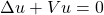on the plane, where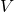is a real, bounded function. The method reduces some questions about solutions to Shrodinger equationon the plane to questions about harmonic functions. Based on a joint work with E.Malinnikova, N.Nadirashvili and F. Nazarov.

### D. Papathanasiou, EIMI

Weighted shifts on directed trees

Weighted backward shifts (unilateral or bilateral) on sequence spaces have been extensively studied and their dynamical properties are well known. We will extend this class of operators by substituting the set of natural numbers or integers by a directed tree and letting the underlying space to be a sequence space on that tree. Motivated by the work of Jablonski, Jung and Stochel, we will define weighted shifts on such spaces and we will discuss when they are hypercyclic or chaotic. We will be interested in relating the corresponding dynamical property to the family of weights of the shift, and the geometry of the tree.

### Stahl-Totik regularity for continuum Schrödinger operators

This talk describes joint work with Benjamin Eichinger: a theory of regularity for one-dimensional continuum Schrödinger operators. For any half-line Schrödinger operator with a bounded potential, we obtain universal thickness statements for the essential spectrum, in the language of potential theory and Martin functions (which will be defined in the talk). Namely, we prove that the essential spectrum is not polar, it obeys the Akhiezer-Levin condition, and moreover, the Martin function at infinity obeys the two-term asymptotic expansion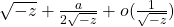as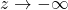. The constant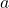in its asymptotic expansion plays the role of a renormalized Robin constant and enters a universal inequality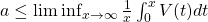. This leads to a notion of regularity, with connections to the exponential growth rate of Dirichlet solutions and limiting eigenvalue distributions for finite restrictions of the operator, and applications to decaying and ergodic potentials.

### Ch. Berg, University of Copenhagen

Indeterminate Hamburger moment problems

A Hamburger moment sequence is of the form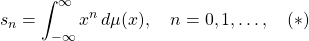where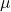is a positive measure on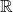. It can be characterized by positive semidefiniteness of the infinite Hankel matrix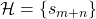. The sequence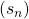can be determinate or indeterminate depending if there is exactly one or several measureson the real line such that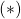holds.

In the talk I will focus on various problems related to the indeterminate case:

• The behaviour of the smallest eigenvalue of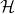.
• The order and type of the functions of the Nevanlinna matrix.
• Behaviour of the infinite matrix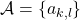of coefficients in the reproducing kernel for the moment problem.

In certain indeterminate cases—but not all—the following infinite matrix equations hold: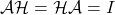.

Some of the results presented are joint work with Ryszard Szwarc, Wrocław.

### E. Liflyand, Bar Ilan University

Wiener algebras and trigonometric series in a coordinated fashion

Let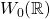be the Wiener Banach algebra of functions representable by the Fourier integrals of Lebesgue integrable functions. It is proven in the paper that, in particular, a trigonometric series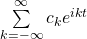is the Fourier series of an integrable function if and only if there exists a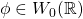such that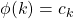,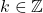. If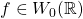, then the piecewise linear continuous function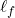defined by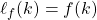,, belongs toas well. Moreover,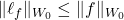. Similar relations are established for more advanced Wiener algebras. These results are supplemented by numerous applications. In particular, new necessary and sufficient conditions are proved for a trigonometric series to be a Fourier series and new properties of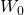are established. This is a joint work with R. Trigub.

### I. Baibulov, EIMI

Eigenfunction expansion for three-body one-dimensional scattering problem

We consider a Schrödinger operator for three particles with pairwise interaction. In the case of d-dimensional particles for d>1 the scattering theory (description of continuous spectrum eigenfunctions or wave operators) is well known, first results are famously due to Faddeev. However, for the case of d=1 the description of generalized eigenfunctions was not complete. We will investigate the one-dimensional case and show how to modify the higher-dimensional results of Faddeev for d=1. At the end of the talk we will address how that approach naturally arises from a microlocal standpoint.

### V. Kapustin, St.Petersburg Department of Steklov Mathematical Institute

The Riemann zeta function and kernels of Toeplitz operators

We modify the Riemann zeta function so that the resulting function becomes an element of the Hardy space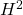in the half-plane where the real part is greater than 1/2. Then we construct the Toeplitz operator, whose kernel contains this function. For the kernel we show how one can construct the Hitt–Sarason representation from their theorem about nearly invariant subspaces.

### A. Pushnitski, King’s College London

The spectrum of some Hardy kernel matrices

A Hardy kernel is an integral kernel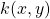in two variables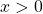and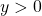which is homogeneous in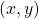of degree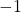. Integral operators on the positive semi-axis with Hardy kernels are explicitly diagonalisable by the Mellin transform. It is however by no means clear how to diagonalise the infinite matrix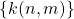which is obtained by restricting a Hardy kernel onto natural numbers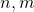. In the talk, I will describe one specific explicit one-parametric family of Hardy kernels k when the spectral analysis ofcan be carried out. This matrix appears in the analysis of composition operators on the Hardy space of Dirichlet series; I will explain this connection at the end of the talk. This is joint work with Ole Brevig (Trondheim) and Kalle Perfekt (Reading).

### G. Rozenblum, Chalmers University of Technology and SPbU

Eigenvalue properties of zero order pseudodifferential operators and an application to a 3D elasticity problem.

We consider a zero order pseudodifferential operator on a compact manifold. Suppose that the operator is polynomially compact. Then there may exist sequences of eigenvalues converging to the points of the essential spectrum. We find their asymptotics, by means of reduction to some negative order operators. The motivating example is the Neumann-Poincare (double layer) operator in 3D elastostatics, where the properties of eigenvlues converging to the points of the essential spectrum are studued.  The general results enable us to separate the contribution of the elastic properties and of the geometry of the body to the eigenvalue asymptotics. Details can be found in https://arxiv.org/abs/2006.10568

### A. Kostenko, University of Vienna

Kirchhoff Laplacians on metric graphs

Laplacians on metric graphs (often called Quantum Graphs) play an important role as an intermediate setting between Laplacians on Riemannian manifolds and Laplacians on graphs and share properties with both. In this talk, we plan to discuss basic spectral properties of Laplacians on metric graphs. The main emphasis will be on graphs with infinitely many vertices and edges, the case which is much less understood.

### R. Romanov, St.Petersburg State University

Discreteness of the spectrum of singular canonical systems.

We are going to provide a criterion for discreteness of the spectrum of canonical systems with one singular end. This criterion simultaneously answers the question of L. de Branges on description of canonical systems corresponding to arbitrary Hermite–Biehler functions. This is a joint work with Harald Woracek (Vienna).

### M. Mitkovski, Clemson University

Uncertainty principles of Paneah-Logvinenko-Sereda type

I will present several new forms of the harmonic analysis uncertainty principle. These new forms can be viewed as a sharpening of the classical Paneah-Logvinenko-Sereda uncertainty principle, in a sense that we impose similar restrictions (sometimes more, sometimes less) on the Fourier support and deduce similar kind of sampling inequalities. I will also present applications of our uncertainty principles to some control and damping problems in linear PDE’s. This is a joint work with W. Green and B. Jaye.

### M. Dorodnyi, EIMI

Operator error estimates for homogenization of the nonstationary Schrödinger-type equations: sharpness of the results.

In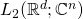, we consider a selfadjoint matrix strongly elliptic second order differential operator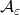, with periodic coefficients depending on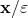. We study the behavior of the operator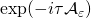for small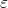. It is proved that, as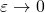, this operator converges to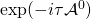in the norm of operators acting from the Sobolev space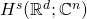(with a suitable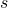) to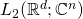. Here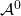is the effective operator with constant coefficients. We prove sharp-order error estimates and study the question about the sharpness of the results with respect to the norm type, as well as to the dependence of estimates on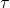. The results are applied to study the behavior of the solution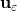of the Cauchy problem for the Schrödinger-type equation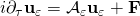.

### V. Peller, St.Petersburg State University

Двойные операторные интегралы и формула следов Лифшица – Крейна

В докладе будут определены двойные операторные интегралы и будут обсуждаться их свойства. Затем мы перейдём к формуле следов Лифшица—Крейна и обсудим, как её можно получить с помощью двойных операторных интегралов. При этом важную роль играет теорема Сёкефальви-Надя и Фойаша об унитарных дилатациях сжатий.

## Asymptotic behavior of Krein system solutions

Krein system is the following system of differential equations: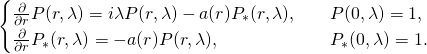We will discuss why functions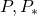are usually called continuous analogues of orthogonal polynomials on the unit circle and consider some of their properties mostly related to behavior of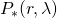as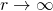.

### V. Sloushch, St.Petersburg State University

Asymptotics of the discrete spectrum appearing in spectral gaps of the discrete Schrodinger operator under decaying sign-definite perturbation

We consider a periodic Schrodinger operator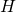on discrete periodic graph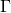in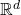. The operatoris perturbed by a sign-definite decaying potentialon the graph;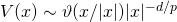,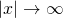,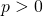. The asymptotics with the large coupling constant of a discrete spectrum of the perturbed operator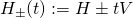,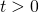is investigated.

### T. Weinmann, St.Petersburg State University

Spectral averaging for rank-one perturbations of self-adjoint operators

Let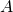be a self-adjoint operator on a Hilbert spaceand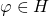. We study the properties of the family of rank-one perturbations ofgiven by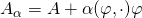, where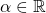In particular, we prove that if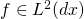, thenfor almost every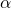(here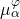denotes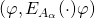) and that in this case the spectral averaging formula holds: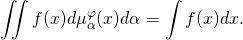### Yu. Belov, St.Petersburg State University

Localization of zeroes for Cauchy transforms and canonical systems

If discrete measure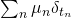is sufficiently small, then the zeroes of Cauchy transform are localized near the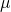. Moreover, it may happen that this holds for any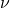such that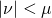. We have found a description of such measures and attraction sets (i.e. subsets ofwhich attract zeroes). We have proved that all attraction sets are ordered by inclusion.

Such measures appear naturaly in the theory of canonical systems of differential equations. They correspond to the canonical systems whose Hamiltonian consists of indivisible intervals accumulating only to the left. Moreover, this correspondence is one-to-one under some additional assumptions. This topic is connected to the problem of density of polynomials and other classical problems in harmonic analysis.

This is joint work with A. Baranov and E. Abakumov.

## Inverse scattering on half line, new results

We solve inverse scattering problem for Schrödinger operators with compactly supported potentials on the half line. We discretize S-matrix: we take the value of the  S-matrix on some infinite sequence of positive real numbers. Using this sequence obtained from S-matrix we recover uniquely the potential by a new explicit formula, without the Gelfand-Levitan-Marchenko equation.